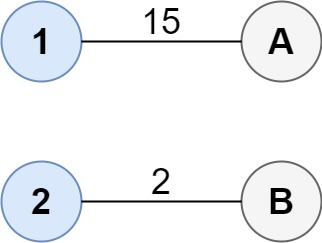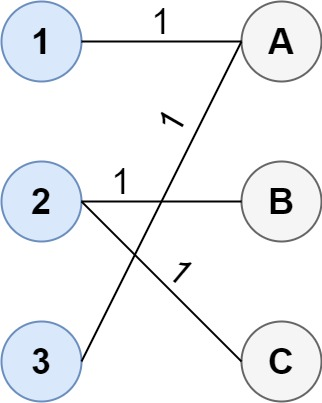Formatted question description: https://leetcode.ca/all/1595.html

# 1595. Minimum Cost to Connect Two Groups of Points (Hard)

You are given two groups of points where the first group has size1 points, the second group has size2 points, and size1 >= size2.

The cost of the connection between any two points are given in an size1 x size2 matrix where cost[i][j] is the cost of connecting point i of the first group and point j of the second group. The groups are connected if each point in both groups is connected to one or more points in the opposite group. In other words, each point in the first group must be connected to at least one point in the second group, and each point in the second group must be connected to at least one point in the first group.

Return the minimum cost it takes to connect the two groups.

Example 1:Input: cost = [[15, 96], [36, 2]]
Output: 17
Explanation: The optimal way of connecting the groups is:
1--A
2--B
This results in a total cost of 17.


Example 2:Input: cost = [[1, 3, 5], [4, 1, 1], [1, 5, 3]]
Output: 4
Explanation: The optimal way of connecting the groups is:
1--A
2--B
2--C
3--A
This results in a total cost of 4.
Note that there are multiple points connected to point 2 in the first group and point A in the second group. This does not matter as there is no limit to the number of points that can be connected. We only care about the minimum total cost.


Example 3:

Input: cost = [[2, 5, 1], [3, 4, 7], [8, 1, 2], [6, 2, 4], [3, 8, 8]]
Output: 10


Constraints:

• size1 == cost.length
• size2 == cost[i].length
• 1 <= size1, size2 <= 12
• size1 >= size2
• 0 <= cost[i][j] <= 100

Related Topics:
Dynamic Programming, Graph

## Solution 1. DP

dp[i][mask] is the state of the first i node in the 1st group being connected, with mask representing which nodes of the 2nd group have been covered already by previous steps in the DFS. (mask[k] = 1 if node k in the 2nd group is already connected).

// OJ: https://leetcode.com/problems/minimum-cost-to-connect-two-groups-of-points/

// Time: O((M * 2^N) * N)
// Space: O(M * 2^N)
class Solution {
int dp = {};
int dfs(vector<vector<int>> &cost, vector<int> &mn, int i, int mask) {
int ans = i < cost.size() ? INT_MAX : 0;
if (i < cost.size()) {
for (int j = 0; j < cost.size(); ++j) {
ans = min(ans, cost[i][j] + dfs(cost, mn, i + 1, mask | (1 << j))); // for nodes in the 1st group, use DP to get the min cost.
}
} else {
for (int j = 0; j < cost.size(); ++j) {
if ((mask & (1 << j)) == 0) ans += mn[j]; // for those unconnected nodes in the 2nd group, pick the min cost to connect to the 1st group
}
}
return dp[i][mask] = ans;
}
public:
int connectTwoGroups(vector<vector<int>>& cost) {
memset(dp, -1, sizeof(dp));
vector<int> mn(cost.size(), INT_MAX); // mn[j] is the minimal cost to connect jth node in the 2nd group to a node in the 1st group
for (int j = 0; j < mn.size(); ++j) {
for (int i = 0; i < cost.size(); ++i) mn[j] = min(mn[j], cost[i][j]);
}
return dfs(cost, mn, 0, 0);
}
};


Java

class Solution {
public int connectTwoGroups(List<List<Integer>> cost) {
int size1 = cost.size(), size2 = cost.get(0).size();
int[][] costMatrix = new int[size1][1 << size2];
for (int i = 0; i < size1; i++) {
for (int j = 0; j < 1 << size2; j++) {
int sum = 0;
for (int k = 0; k < size2; k++) {
if ((j & (1 << k)) > 0)
sum += cost.get(i).get(k);
}
costMatrix[i][j] = sum;
}
}
int[][] dp = new int[size1][1 << size2];
for (int i = 1; i < size1; i++)
Arrays.fill(dp[i], Integer.MAX_VALUE);
dp = costMatrix;
for (int i = 1; i < size1; i++) {
for (int j = 1; j < 1 << size2; j++) {
for (int k = 0; k < size2; k++)
dp[i][j | (1 << k)] = Math.min(dp[i][j | (1 << k)], dp[i - 1][j] + cost.get(i).get(k));
int rest = (1 << size2) - 1 - j;
for (int k = rest; k >= 1; k = rest & (k - 1))
dp[i][j | k] = Math.min(dp[i][j | k], dp[i - 1][j] + costMatrix[i][k]);
}
}
return dp[size1 - 1][(1 << size2) - 1];
}
}